<68r>

## De Solutione Problematum per Motum

Ne hujusmodi operationes obscuræ nimis evadant, Lemmata 6 sequētia brevitèr ideo non demonstrati|a| præmittam.

Lemma 1. Si corpus A in circumferentiâ circuli vel sphæræ ADCE moveatur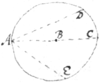versus ejus centrum B: velocitas ejus ad unaquæ circumferentiæ puncta D, C, E, est ut cordæ AD, AC, AE, ductæ a Corpore A ad ista puncta D, C, E.

Lemma 2. Sit △ ADC similis △AEC, etsi non sunt in eodem plano. Inquam,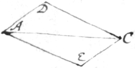Si tria Corpora a puncto A, primum ad D, secundum ad E, tertium ad C, uniformiter et in æqdua|lib| tempore|i|bus moveantur: Motus tertij componetur ex motibus primi et scdi.

Notetur, quòd hic per corpus intelligitur ejus centrum gravitatis, vel aliquod ejus punctum.

Lemma 3. Omnia puncta corporis parallelismum servantis æqualiter moventur.

Lemma 4. Si corpus solo motur circulari circa axim quemvis rotetur; motus omnium ejus punctorum sunt ut distantiæ ab isto axi. Et hi duo motus simplices vocentur.

Lemma 5. Si motus corporis consideretur ut mixtus e motibus simplicibus: motus omnium ejus punctorum componetur ex motibus eorum simplicibus, eo modo quo motus ab A ad C, in Lem̄ate 2do componitur ex motibus ab A ad D et E.

Nota. qd motus quilibet ad unum horum 3ū casuum reduci poterit. Et in casu tertio, linea quævis pro axe, (vel si linea aut plana superficies move{t}|a|tur in plano, quodvis punctum istius plani pro centro) motus assumi potest.

Lemma 6. Sint AE, AH, lineæ motæ et continuò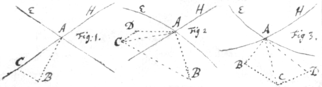secantes; Ducantur AB, AD, AC, BC {sic}, CD. Dico quòd, datis quæ requirantur ad proportiones et positiones harum quinque linearum AB, AD, AC, CB, DC, determinandas; illæ designent proportiones et positiones h{o}rum quin motu{illeg}|{um}|, viz: puncti a|A| in lineâ AE fixi et versus B moventis, puncti A in lineâ AH fixi et versus D moventis, Puncti {illeg} intersectionis A in plano ABCD {est} moventis versus C, (lineæ enim 5 istæ semper sunt in eodem plano \etsi AE AH non sunt/), Puncti intersectionis A in lineâ AE moventis secundum ordinem literarum C, B, et Puncti intersectionis A in lineâ AH moventis secundum ordinem C. D.

{Nota}. Quod linea recta {ut} \positionem/ mot{ûs} curvi{æ} \motûs curvi/ eandem designare dicitur

Nota. Quòd cùm linea recta tang{i}t|en|s curvam motu descriptam (ut AB, AD, AC)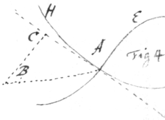vel istæ tangenti parallela est (ut CB, CD,), {illeg} dicitur designare positionem istius motûs in puncto contactûs.

Nota etiam qd lineâ AH quiescente (ut in Fig 1, et 4), punctum D et A coincident et puctum {sic} C in lineâ AH, modò sit rectâ|a| (Fig 1,), alitèr in ejus tangente AC (fig 4) reperietur.

Prop 1. Ducere Tangentem ad Ellipsin.

Sit ACB filum per quod ellipsis describi solet, et CE Tangens. Cùm filum AC augetur eâdem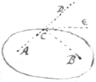velocitate quâ BC diminuitur, i:e: C habet eandem velocitatem versus D et B; eit|r|it <DCE=ECB.Per Lem 1. Idem de reliquis Conicis intelligatur.

Prop 2. Ducere Tangentem ad Conchoïden

Sint GLC, ALF, GAE, regulæ quibus concha describi solet: fiat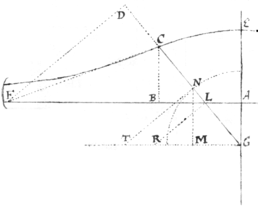GT∥AF⊥CB=∥MN: et NG=CL⊥TN∥RL. |Et|, Cùm æqualitas proportionalitate simplicior est, ponatur lineam CB=NM esse æqualem velocitati puncti C versus B, vel puncti N versus M. Erit NT=motui circulari puncti N circa G rotantis versus T, (Lem 1): et LR=Motui circulari puncti L in lineâ GL fixi, circa G (Lem 4): et LG = velocitati motui puncti intersectionis L (i:e: velocitati puncti C) a puncto G, sive versus punctum D in lineâ C G moventis, (Lem 6). Iam cum habeatur duplex \duplex/ velocitas puncti C versus {illeg} viz CB versus B et LG versus D, fiat FD⊥DC=LG: Et motus puncti C erit in lineâ FC, Diametro nempe circuli transientis per puncta L|C|DBF, (Lem 1): Quæ proinde Conchi|a|m tangit in puncto C.

<68v>

Prop 3. Invenire punctum C distinguens concavam a convexâ Conchæ portione.

Iis in priori propositione suppositis: Fiat △us GFH similis △o GNT sive LBC: et DF⊥FR∥=HK=2GL; Iungātur F, K, et fiat KP∥FD. Si Linea DF solum motum Parallelum per CD vel FR directum haberet, (quia CD=GL) motus omnium ejus punctorum esset FR, (Lem 3): Et si solum motum circularem circa centrū G haberet, motus puncti F, in istâ lineâ DF fixi, esset FH, (Lem 4). At motus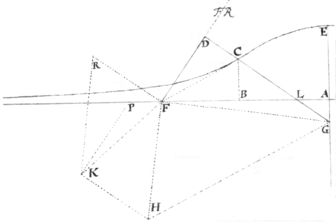istius F ex istis duobus componitur, proinde erit FK, (Lem 5., 2.) et motus puncti intersectionis F per lineas AF, DF, fi|a|cti, et in AF moventis, erit FP (Lem 6). Iam si linea CF Concham tangit in puncto quæsito C, facilè deprehendatur motum puncti intersectionis F esse nullum; proinde P et F coincidere; sive DF et FK in directum jacere; et △GDF, FKH esse similes.

Quæ ut cal|c|ulo subjiciantur, fiat AG=b. CL=c. CB=y. tum, $BL=\sqrt{cc-yy}$ . $2GL=\frac{2bc}{y}=HK$ . $LD=\frac{cb+cy}{y}$ . $DF=\frac{cb+cy}{\sqrt{cc-yy}}$ . Et|c|. $\sqrt{cc-yy}:y\colon\colon BL:CB\colon\colon GF:FH\colon\colon DF:KH\colon\colon \frac{cb+cy}{\sqrt{cc-yy}}:\frac{2bc}{y}\colon\colon by+yy:2b\sqrt{cc-yy}$ . Quare, $2bcc-2byy=byy+{y}^{3}$ . sive ${y}^{3}+3byy\ast -2bcc=0$ . < insertion from f 69r > Equatio ${y}^{3}+3byy\ast -2bcc=0$ , priùs inventa, ita resolve, fac $Ao=\frac{8{c}^{4}}{27{b}^{3}}$ . $af=c=AE$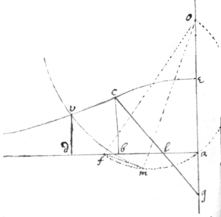duc {of} et cum diametro of describe circulum fmao, in quo inscribe fm=b=AG. et cum radio om, fac circulum mv, et et a pūcto intersectionis v d duc vd⊥ad. erit 3b∶2c∷VD∶Gl. unde datur punctum c. < text from f 68v resumes >

Vsus autem hujus methodi (ut intelligo) præcipuus est in lineis Mechanicis, ubi deficit Algebraica calculatio. Exempli gra, Tangens Quadratrici ità ducetur.

Sint AG, MC regulæ quibus uniformita|è|r motis Quadratrix describi intelligitur. Et CBvocetur motus puncti C in lineâ CM fixi et versus B moventis; tum arcus GK erit motus puncti G cira {sic} A rotantis, \(sup)/ et arcus CL erit motus puncti C in lineâ AG fixi et circa G rotantis, (lem 4). Quare ({illeg} si fiat CL=CD⊥AG∥DF, et BF∥CM.) motus puncti intersectionis C \in planò AEK/ erit CF (Lem 6), quæ proinde {illeg}|Q|uadr tangit in C.

<69r> <70r>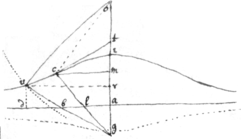If vce is a Conchoïdes, g its pole, &c: ga=b. ae=lc=vb=c. ma=y & c ye point betwixt its convexity & concavity, yn is ${y}^{3}+3byy\ast -2bcc=0$ . (see pag: 259. lin: 10.). Wch Equation hath one affirmative roote (ma) referred to ye point c. and two negative rootes whereof ye greater is referred to ye lower Conch, & ye lesser (I thinke) uselesse{illeg} to this question.

The rootes of this Equation may bee thus found by ye hel{f}|p|e of the described Conch & a circle. viz: Suppose ga=b, ae=lc=vb=c. ma=y. (as before) a{illeg}|{o}|=s. oc=r. And thereby may bee found this Equation $\frac{{y}^{3}\overline{+rr-ss+bb-cc}×yy-2bccy-bbcc}{2s+2b}=0$ , to bee compared wth ye former, (see pag 261 lin 20). But their rootes cannot become equall by reason of their third the termes. Therefore I alter ye rootes of ye first equation, as, suppose I make y=z−b. Then is z3∗−3bbz+2b3−2bcc=0. To bee compared wth ye 2d equation: wch cannot yet bee done wthout a contradiction, there being but two {illeg} unknowne quantitys, {illeg} r & s to bee found by three Equations resulting from ye comparison of their 2d 3d & 4th termes. But if I make $z=\frac{3bbx}{4cc-4bb}$ $z=\frac{4ccx-4bbx}{3bb}$ , & substitute this valor into its pal|la|ce in ye precedent Equation, the result is ${x}^{3}\ast \frac{-27{b}^{6}x}{16{c}^{4}-32bbcc+16{b}^{4}}\frac{-27{b}^{7}}{32{c}^{4}-64bbcc+32{b}^{4}}=0$ . The termes of wch being compared with ye termes of ye 2d Equation ye 3d or 4th give $\frac{2bbc}{2s+2b}=\frac{27{b}^{6}}{16{c}^{4}-32bbcc+16{b}^{4}}$ . Or 16c6−32bbc4+16b4cc=27b6+27b5 s. & s=16c6{illeg}bbc4+6b4 $s=\frac{16{c}^{6}}{27{b}^{5}}-\frac{32{c}^{4}}{27{b}^{3}}+\frac{16cc}{27b}-b=ao$ . Their second termes give $\frac{rr-ss+bb-cc}{2s+2b}=0$ . Or $r=\sqrt{ss+cc-bb}=ov$ . Therefore from ye intersection point v (made by ye Conch & a circle whose radius is ov & center o) let fall ve|d|⊥ad; & ar=x\=vd,/ is ye roote of ye 2d & last equation. Which being found make $y=\frac{4ccx-4bbx}{3bb}-b=am$ . Which was to /bee done.\Prop 1. Suppose ab=x. bd=y⊥ab. And yt ye nature of ye line addc is such yt ye valor of y is rationall & consists not \of no fractions in whose denominator x is, or else wholy of {sic}/ of such fractions in whose denominators x is, \but not/ {illeg} of divers dimensions: If I then multiply ye valor of y by x, & divide \{uni}/ each terme of yt valor by so many units as {illeg}|it| hath dimensions in yt {it} \terme/; ye result shall signifie ye area abd of ye afforesaide line addc.

As for example. If y=1, or y=x, or y=xx, or x3, or x4 &c yn ye area abd is $\frac{1xx}{1}$ , or $\frac{xxx}{2}$ , or $\frac{xxxx}{3}$ , or $\frac{{x}^{3}xx}{4}$ , or $\frac{{x}^{4}xx}{5}$ &c: so if $y=\frac{a}{b}$ , or $y=\frac{ax}{b}$ , or $\frac{axx}{b}$ &c: yn ye area abd is $\frac{ax}{b}$ , $\frac{axx}{2b}$ , $\frac{a{x}^{3}}{3b}$ &c. In like manner if $y=\frac{a}{xx}$ , or $y=\frac{a}{{x}^{3}}$ , or $y=\frac{a}{{x}^{4}}$ &c yn $\frac{a}{±x}=\frac{-a}{x}$ , or $\frac{a}{-2xx}=$ {illeg}, or $\frac{a}{-3{x}^{3}}$ ={illeg}, &c: is ye area abd (as is by others demontrated {sic}). [so if $\frac{a}{x}=y$ . yn $\frac{a}{0}=\frac{a{x}^{0}}{0}$ is ye area abd; viz: tis infinite.]. Lastly if $y=1+x+axx-b{x}^{3}-\frac{{x}^{4}}{d}+\frac{c{x}^{15}}{e+f}$ &c. ye area abd is $x+\frac{1}{2}xx+\frac{a{x}^{3}}{3}-\frac{b{x}^{4}}{4}-\frac{{x}^{5}}{5d}+\frac{c{x}^{6}}{16e+16f}$ &c. For ye area abd is compounded of those areas wch are related to & generated by those quantitys of wch ye valor of y is compounded. & wt those areas are appeare by ye former example. ({n}|N|ote yt Parabolicall & Hyperbolicall (i:e: negativ & \(in respect of bd,)/ affirmative & negative) areas (thus considered) cannot compound any 3d area, because they are not on ye same side of ye line bd.)

Prop 2. {illeg} $\frac{aa}{b+x}=$ {illeg} $\frac{aa}{b}-\frac{aax}{bb}+\frac{aaxx}{{b}^{3}}-\frac{aa{x}^{3}}{{b}^{4}}+\frac{aa{x}^{4}}{{b}^{5}}-\frac{aa{x}^{5}}{{b}^{6}}+\frac{aa{x}^{6}}{{b}^{7}}$ &c. For these termes are r $\frac{aa}{b+x}$ . aa. aab+aax. aabb+2aabx+aaxx. aab3+3aabbx+3aabxx+aax3, & which termes may bee thus ordered This lest appeares by multiplying both parts by b+x

<70v>

$\begin{array}{rrrrrr}\frac{aa}{b+x}.& aa.& aab.& aabb.& aa{b}^{3}.& aa{b}^{4}.& \phantom{}\\ \phantom{}\phantom{}aax.& 2aabx.& 3aabbx.& 4aa{b}^{3}x.& \text{&c}:\\ \phantom{}\phantom{}\phantom{}aaxx.& 3aabxx.& 6aabbxx.& \phantom{}\\ \phantom{}\phantom{}\phantom{}\phantom{}aa{x}^{3}.& 4aab{x}^{3}.& \phantom{}\\ \phantom{}\phantom{}\phantom{}\phantom{}\phantom{}aa{x}^{4}.& \phantom{}\end{array}$

Or by supplying ye vacant places

$\begin{array}{rrrrrr}\frac{aa}{b+x}.& 1×aa.& 1×aab.& 1×aabb.& 1×aa{b}^{3}.& 1×aa{b}^{4}.\\ \phantom{}& 0×\frac{aax}{b}.& 1×aax.& 2×aabx.& 3×aabbx.& 4×aa{b}^{3}x.\\ \phantom{}& 0×\frac{aaxx}{bb}.& 0×\frac{aa{x}^{2}}{b}.& 1×aaxx.& 3×aabxx.& 6×aabbxx.\\ \phantom{}& 0×\frac{aa{x}^{3}}{b}.& 0×\frac{aa{x}^{3}}{bb}.& 0×\frac{aa{x}^{3}}{b}.& 1×aa{x}^{3}.& 4×aab{x}^{3}.\\ \phantom{}& 0×\frac{aa{x}^{4}}{{b}^{4}}.& 0×\frac{aa{x}^{4}}{{b}^{3}}.& 0×\frac{aa{x}^{4}}{bb}.& 0×\frac{aa{x}^{4}}{b}.& 1×aa{x}^{4}.\end{array}$

Now to reduce ye first terme $\frac{aa}{b+x}$ to ye same forme wth ye rest, I consider in what progressions ye numbers prefixed to these termes proceede, & find ym to bee {illeg} such yt {ye} any number added to ye number above it is equall to ye number following it. Whence any termes may bee found wch are wanting, as in ye annexed Table. Also any terme, {illeg}|t|o wch these numbers are prefixed, being multiplyed by b produceth ye following \litterall/ terme. Or {ye} ye higher terme multiplyed by $\frac{x}{b}$ , divided by b producceth ye lower terme. As in ye following table

$\begin{array}{rrrrrrrrrrrrr}1.& 1.& 1.& 1.& 1.& 1.& 1.& 1.& 1.& 1.& 1.& 1.& \\ -4.& -3.& -2.& -1.& 0.& 1.& 2.& 3.& 4.& 5.& 6.& 7.& \\ 10.& 6.& 3.& 1.& 0.& 0.& 1.& 3.& 6.& 10.& 15.& 21.& \\ -20.& -10.& -4.& -1.& 0.& 0.& 0.& 1.& 4.& 10.& 20.& 35.& \text{&c}\\ 35.& 15.& 5.& 1.& 0.& 0.& 0.& 0.& 1.& 5.& 15.& 35.& \\ -56.& -21.& -6.& -1.& 0.& 0.& 0.& 0.& 0.& 1.& 6.& 21.& \\ 84.& 28.& 7.& 1.& 0.& 0.& 0.& 0.& 0.& 0.& 1.& 7.& \end{array}$

$\begin{array}{cc}\begin{array}{ccccccccccccccccc}\frac{aa}{\overline{b+x}{\right)}^{3}}& .& \frac{aa}{\overline{b+x}{\right)}^{2}}& .& \frac{aa}{b+x}& .& aa& .& aa×b+x& .& {a}^{2}×\overline{b+{x}^{2}}& .& {a}^{2}×\overline{b+{x}^{3}}& .& {a}^{2}×\overline{b+{x}^{4}}& .& \\ =& & =& & =& & =& & =& & =& & =& & =& & \\ +\frac{aa}{{b}^{3}}& .& +\frac{aa}{bb}& .& +\frac{aa}{b}& .& +aa& .& +aab& .& +aabb& .& +aa{b}^{3}& .& +aa{b}^{4}& .\\ -\frac{3aax}{{b}^{4}}& .& \frac{-2aax}{{b}^{3}}& .& \frac{-aax}{bb}& .& \frac{0×aax}{b}& .& +aax& .& +2aabx& .& +3aabbx& .& +4aa{b}^{3}x& .& \\ \frac{+6aa{x}^{2}}{{b}^{5}}& .& \frac{+3aa{x}^{2}}{{b}^{4}}& .& \frac{+aaxx}{{b}^{3}}& .& \frac{0×aa{x}^{2}}{{b}^{2}}& .& \frac{0×aaxx}{b}& .& +aaxx& .& +3aabxx& .& +6aabb{x}^{2}& .& \text{&c}\\ \frac{-10aa{x}^{3}}{{b}^{6}}& .& \frac{-4aa{x}^{3}}{{b}^{5}}& .& \frac{-aa{x}^{3}}{{b}^{4}}& .& \frac{0×aa{x}^{3}}{{b}^{3}}& .& \frac{0×aa{x}^{3}}{bb}& .& \frac{0×aa{x}^{3}}{b}& .& +aa{x}^{3}& .& +4aab{x}^{3}& .& \\ \frac{+15aa{x}^{4}}{{b}^{7}}& .& \frac{+5aa{x}^{4}}{{b}^{6}}& .& \frac{+aa{x}^{4}}{{b}^{5}}& .& \frac{0×aa{x}^{4}}{{b}^{4}}& .& \frac{0×aa{x}^{4}}{{b}^{3}}& .& \frac{0×aa{x}^{4}}{bb}& .& \frac{0×aa{x}^{4}}{b}& .& +aa{x}^{4}& .& \\ \text{&c}& .& \text{&c}& .& \text{&c}& .& \text{&c}& .& \text{&c}& .& \text{&c}& .& \text{&c}& .& \text{&c}& .& \end{array}& \begin{array}{l}\text{Whence it appeares}{y}^{t}\\ \frac{aa}{b+x}=\frac{aa}{b}-\frac{aax}{bb}+\frac{aaxx}{{b}^{3}}-\frac{aa{x}^{3}}{{b}^{4}}& \text{&c. Or}{y}^{t}\\ \frac{aa}{b-x}=\frac{aa}{b}+\frac{aax}{bb}+\frac{aaxx}{{b}^{3}}+\frac{aa{x}^{3}}{{b}^{4}}& \text{&c. Or}{y}^{t}\\ \frac{aa}{b+x}=\frac{aa}{x}-\frac{aab}{xx}+\frac{aabb}{{x}^{3}}-\frac{aa{b}^{3}}{{x}^{4}}& \text{&c. Or}{y}^{t}\\ \frac{aa}{bb+2bx+xx}=\frac{aa}{bb}-\frac{2a2x}{{b}^{3}}+\frac{3aaxx}{{b}^{4}}& \text{&c.}\\ \\ \\ \\ \end{array}\end{array}$

Prop: 3d. If ab=x. y=db⊥ab⊥ac. & aa (fa=b) &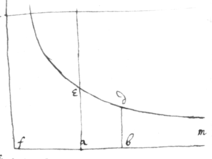$\frac{aa}{b+x}=y=\left(prop2\right)\frac{aa}{b}-\frac{aax}{bb}+\frac{aaxx}{{b}^{3}}-\frac{aa{x}^{3}}{{b}^{4}}+\frac{aa{x}^{4}}{{b}^{5}}$ &c. Then (by prop 1), $\frac{aax}{b}-\frac{aaxx}{2bb}+\frac{aa{x}^{3}}{3{b}^{3}}-\frac{aa{x}^{4}}{4{b}^{4}}+\frac{aa{x}^{5}}{5{b}^{5}}-\frac{aa{x}^{6}}{6{b}^{6}}$ &c: is abde, ye area of ye Hyperbola. So if $\frac{aa}{b-x}=y$ : &c. In like manner if $\frac{aa}{bb+2bx+xx}=y=\left(prop2\right)\frac{aa}{bb}-\frac{2aax}{{b}^{3}}+\frac{3aaxx}{{b}^{4}}-\frac{4aa{x}^{3}}{{b}^{5}}$ &c. Then (by prop 1) $\frac{aax}{bb}-\frac{aaxx}{{b}^{3}}+\frac{aa{x}^{3}}{{b}^{4}}-\frac{aa{x}^{4}}{{b}^{5}}&c=\left(prop2\right)\frac{aa}{b}-\frac{aa}{b+x}$ is ye area abde. (wch may also thus appeare viz: if fb=b+x=z. yn $\frac{aa}{bb+2bx+xx}=\frac{aa}{zz}=y$ . Therefore (prop 1) ye area $dbm=\frac{aa}{z}=\frac{aa}{b+x}$ . & $eam=\frac{aa}{b}$ . so yt $eadb=\frac{aa}{b}-\frac{aa}{b+x}$ ). And so of ye rest. As if $\frac{{x}^{3}}{aa+bx+{x}^{2}}=y=\left(prop{2}^{d}\right)\frac{{x}^{3}}{aa}\frac{-b{x}^{4}-{x}^{5}}{{a}^{4}}+\frac{bb{x}^{5}+2b{x}^{6}+{x}^{7}}{{a}^{6}}\frac{-bbb{x}^{6}-3bb{x}^{7}-3b{x}^{8}-{x}^{9}}{a8}$ &c. The area abde is $\frac{4{x}^{4}}{aa}-\frac{5b{x}^{5}-6{x}^{6}}{{a}^{4}}\frac{+6bb{x}^{6}+14b{x}^{7}+8{x}^{8}}{{a}^{6}}\frac{-7{b}^{3}{x}^{7}-24bb{x}^{8}-27b{x}^{9}-10{x}^{10}}{{a}^{8}}$ &c.

Prop 4th. If $\frac{a}{b}{x}^{n}={y}^{m}$ . n & m being numbers yt signifie ye dimensions of x & y: Then is, $\frac{mx}{m+n}\sqrt{m:\frac{a}{b}{x}^{n}}=\frac{mxy}{m+n}$ . The area abde, \if n is affirmative/ or mbd \iif n is negave {sic}/ of ye line edm. Prop: 5t. If $y=a{x}^{m}+b{x}^{n}$ . {illeg} yn $\frac{xxa{x}^{m}}{m+1}+\frac{xb{x}^{n}}{n+1}=abde$ .

Prop 4th. To find two or three intermediate termes in ye above mentioned table of numerall

 progressions, I observe yt those progressions are of this nature viz a. a. a a. a. b. b+c. b+2c. b+3c. b+4c. And yt ye summe of any terme & ye terme above it is equall to d. d+e. d+2e+f. d+3e+ef. d+4e+6f. ye terme following it at such a|the| distance at|of| ye termes in ye sd numerall g. g+h. g+2h+i. g+3h+3i+k. g+4h+6i+4k. {illeg}re one from ye other table. Suppose I would find ye meane l. l+m. l+2m+n. l+3m+3n+p. l+4m+6n+4p+q. r. r+s. r+2s+t. r+3s+3t+v. r+4s+6t+4v+w.
<71r>

termes in ye 3d progression

 3. *. 1. *. 0. *. 0. *. 1. *. 3. d−4e+10f. d−3e+6f. d−2e+3f. d−e+f d. d+e d+2e+f. d+3e+f d+4e+6f. d+5e+10f. d+6e+15f.

I compare ye termes of yt progression & of ye correspondent litterall progression & find d=0=2e+f. 4e+6f=1. subduct 4e+2f=0, Or 12e+6f=0 from 4e+6f=1. & ye rest is 4f=1. Or −8e=1. wch termes being found viz d=0. $e=\frac{-1}{8}$ . $f=\frac{1}{4}$ . ye progression must be $3·\frac{9}{8}·1·\frac{3}{8}·0·\frac{-1}{8}·0·\frac{3}{8}·1·\frac{15}{8}·3·$ &c. Hence may be deduced this table viz $\begin{array}{rrrrrrrrrrrrrrrrrr}1.& 1.& 1.& 1.& 1.& 1.& 1.& 1.& 1.& 1.& 1.& 1.& 1.& 1.& 1.& 1& 1.& \\ -3.& \frac{-5}{2}.& -2.& \frac{-3}{2}.& -1.& \frac{-1}{2}.& 0.& \frac{1}{2}.& 1.& \frac{3}{2}.& 2.& \frac{5}{2}.& 3.& \frac{7}{2}.& 4.& \frac{9}{2}.& 5.& \\ 6.& \frac{35}{8}.& 3.& \frac{15}{8}.& 1.& \frac{3}{8}.& 0.& \frac{-1}{8}.& 0.& \frac{3}{8}.& 1.& \frac{15}{8}.& 3.& \frac{35}{8}.& 6.& \frac{63}{8}.& 10.& \\ -10.& \frac{-105}{16}.& -4.& \frac{-35}{16}.& -1.& \frac{-5}{16}.& 0.& \frac{1}{16}.& 0.& \frac{-1}{16}.& 0.& \frac{5}{16}.& 1.& \frac{35}{16}.& 4.& \frac{105}{16}.& 10.& \text{&c}\\ 15.& \frac{1155}{128}.& 5.& \frac{315}{128}.& 1.& \frac{35}{128}.& 0.& \frac{-5}{128}.& 0.& \frac{3}{128}.& 0.& \frac{-5}{128}.& 0.& \frac{35}{128}.& 1.& \frac{315}{16}.& 5.& \\ -21.& \frac{-3003}{256}.& -6.& \frac{-693}{256}.& -1.& \frac{-63}{256}.& 0.& \frac{7}{256}.& 0.& \frac{-3}{256}.& 0.& \frac{3}{256}.& 0.& \frac{-7}{256}.& 0.& \frac{63}{256}.& 1.& \\ +28.& \frac{15015}{1024}.& 7.& \frac{3003}{1024}.& 1.& \frac{231}{1024}.& 0.& \frac{-21}{1024}.& 0.& \frac{7}{1024}.& 0.& \frac{-5}{1024}.& 0.& \frac{7}{1024}.& 0.& \frac{-21}{1024}.& 0.& \\ &c:& &c:& &c:& &c:& &c:& &c:& &c:& &c:& &c:& &c:& &c:& &c:& &c:& &c:& &c:& &c:& &c:& \end{array}$

Note yt ye progression $\begin{array}{ccccccc}1.& \frac{1}{2}.& \frac{-1}{8}.& \frac{1}{16}.& \frac{-5}{128}.& \frac{7}{256}& &c:\end{array}$ may bee deduced from hence $\begin{array}{ccccccccccccccc}1& ×& 1& ×& -1& ×& -3& ×& -5& ×& -7& ×& -9& ×& -11\\ 1& ×& 2& ×& 4& ×& 6& ×& 8& ×& 10& ×& 12& ×& 14\end{array}$ &c & one intermediate terme given ye rest are easily deduced thence.

In like manner if I would find two meanes twixt every terme of yt numerall progress I compare ye numerall & correspondent litterall progressions, suppose in ye 3d progression.

 1· *· *· 0· *· *· 0· *· *· 1· d−3e+6f. d−2e+3f. d−e+f. d. d+e d+2e+f. d+3e+3f. d+4e+6f. d+5e+10f. d+63+15f. &c.

And find yt /d=0=3e+3f.\ & {6e+5f=} 6f−3e=1. To wch adding 3e+3f=0, or −6f−6e=0. ye result is 9f=1, or −9e=1. So yt ye progression must bee $\begin{array}{ccccccccccccc}1.& \frac{5}{9}.& \frac{2}{9}.& 0.& \frac{-1}{9}.& \frac{-1}{9}.& 0.& \frac{2}{9}.& \frac{5}{9}.& 1.& \frac{14}{9}.& \frac{20}{9}.& 3.\end{array}$ &c. Hence may bee composed this $\begin{array}{cccccccccccccc}\text{Table}& 1.& 1.& 1.& 1.& 1.& 1.& 1.& 1.& 1.& 1.& 1.& 1.& 1.\\ & 0.& \frac{1}{3}.& \frac{2}{3}.& 1.& \frac{4}{3}.& \frac{5}{3}.& 2.& \frac{7}{3}.& \frac{8}{3}.& 3.& \frac{10}{3}.& \frac{11}{3}.& 4.\\ & 0.& \frac{-1}{9}.& \frac{-1}{9}.& 0.& \frac{2}{9}.& \frac{5}{9}.& 1.& \frac{14}{9}.& \frac{20}{9}.& 3.& \frac{35}{9}.& \frac{44}{9}.& 6.\\ & 0.& \frac{5}{81}.& \frac{4}{81}.& 0.& \frac{-4}{81}.& \frac{-5}{81}.& 0.& \frac{14}{81}.& \frac{40}{81}.& 1.& \frac{140}{81}.& \frac{220}{81}.& 4.\\ & 0.& \frac{-10}{243}.& \frac{-7}{243}.& 0.& \frac{+5}{243}.& \frac{+5}{243}.& 0.& \frac{-7}{243}.& \frac{-10}{243}.& 0.& \frac{25}{243}.& \frac{110}{243}.& 1.\end{array}$ Note yt this progression viz $\begin{array}{ccccccccccccc}1& ×& 1& ×& -2& ×& -5& ×& -8& ×& -11& ×& -14\\ 1& ×& 3& ×& 6& ×& 9& ×& 12& ×& 15& ×& 18\end{array}$ &c gives ye second term $1+\frac{1}{3}-\frac{1}{9}+\frac{5}{81}-\frac{10}{243}+\frac{22}{729}$ &c. & this $\begin{array}{ccccccccccccc}1& ×& 2& ×& -1& ×& -4& ×& -7& ×& -10& ×& -13\\ 1& ×& 3& ×& 6& ×& 9& ×& 12& ×& 15& ×& 18\end{array}$ &c gives ye third terme. Also this progression $\begin{array}{ccccccccccccccc}1& ×& 4& ×& 1& ×& -2& ×& -5& ×& -8& ×& -11& ×& -14\\ 1& ×& 3& ×& 6& ×& 9& ×& 12& ×& 15& ×& 18& ×& 21\end{array}$ &c. gives ye 4th.

And in Generall if ye 2d quantity of any terme is $\frac{x}{y}$ , {illeg} this progression gives all ye rest viz $\begin{array}{ccccccccccccccc}1& ×& x& ×& \overline{x-y}& ×& \overline{x-2y}& ×& \overline{x-3y}& ×& \overline{x-4y}& ×& \overline{x-5y}& ×& \overline{x-6y}\\ 1& ×& y& ×& 2y& ×& 3y& ×& 4y& ×& 5y& ×& 6y& ×& 7y\end{array}$ &c. And $\frac{x}{y}$ is ever given by supposition for it signifieth ye distance of ye terme from 1.0.0.0.

$\begin{array}{}\begin{array}{c}\text{Note also}{y}^{t}\text{any}\\ \text{of these progressions}\\ {w}^{th}\text{their intermediate}\\ \text{termes}\end{array}\begin{array}{ccccccc}1.& 1.& 1.& 1.& 1.& 1.& \\ 0.& 1.& 2.& 3.& 4.& 5.& \\ 0.& 0.& 1.& 3.& 6.& 10.& \text{&c.}\\ 0.& 0.& 0.& 1.& 4.& 10.& \\ 0.& 0.& 0.& 0.& 1.& 5.& \\ 0.& 0.& 0.& 0.& 0.& 1.& \\ 0.& 0.& 0.& 0.& 0.& 0.& \end{array}\begin{array}{c}\text{may bee designed}\\ \text{by Geometricall}\\ \text{lines}\end{array}\begin{array}{l}1=y.\\ x=y.\\ xx-x=2y.\\ {x}^{3}-3xx+2x=6y.\\ {x}^{4}-6{x}^{3}+11xx-6x=24y.\\ {x}^{5}-10{x}^{4}+35{x}^{3}-50{x}^{2}+24x=120y.\\ {x}^{6}-15{x}^{5}+85{x}^{4}-225{x}^{3}+274xx-120x=720y.\end{array}\end{array}$ In wch x signifieth ye distance of any terme from ye first 1.0.0.0.0.0. & y is the quantity of yt terme.

Prop 5t.

<72r>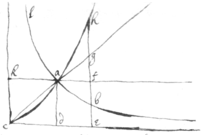If lab is an Hyperbola; cde, ck its Asymptotes, a its vertex, & cag its axis; <ckd=2<kca=<kcd=<cda=<ceb \if adef is a square & he∥ad/ & cd=1, |&,| de=x. yn $be=\frac{1}{1+x}$ . If also, {illeg}|e|f=1. eg=1+x. eh=1+2x+x &c: (a|t|he progression continued is 1+3x+3xx+x3. 1+4x+6x2+4x3+x4. 1+5x+10x2+10x3+5x4+x5 &c). Then, shall the areas of those lines proceede{t} in this progression. *=adeb. x=adef. $x+\frac{xx}{2}=adeg$ . $adeh=x+\frac{2xx}{2}+\frac{{x}^{3}}{3}$ . $x+\frac{3xx}{2}+\frac{3{x}^{3}}{3}+\frac{{x}^{4}}{4}$ . $x+\frac{4xx}{2}+\frac{6{x}^{3}}{3}+\frac{4{x}^{4}}{4}+\frac{{x}^{5}}{5}$ &c. As in this table. In wch ye first area is also inserted. The composition of wch table may be $\begin{array}{cc}\begin{array}{rrrrrrrrrrr}x& ×& 1.& 1.& 1.& 1.& 1.& 1.& 1.& 1.& 1.\\ \frac{xx}{2}& ×& -1.& 0.& 1.& 2.& 3.& 4.& 5.& 6.& 7.\\ \frac{{x}^{3}}{3}& ×& 1.& 0.& 0.& 1.& 3.& 6.& 10.& 15.& 21.\\ \frac{{x}^{4}}{4}& ×& -1.& 0.& 0.& 0.& 1.& 4.& 10.& 20.& 35.\\ \frac{{x}^{5}}{5}& ×& 1.& 0.& 0.& 0.& 0.& 1.& 5.& 15.& 35.\\ \frac{{x}^{6}}{6}& ×& -1.& 0.& 0.& 0.& 0.& 0.& 1.& 6.& 21.\\ \frac{1}{7}{x}^{7}& ×& 1.& 0.& 0.& 0.& 0.& 0.& 0.& 1.& 7.\\ & & =& =& =& =& & & & & \\ & & adeb.& adef.& adeg.& adeh.& \text{&c}\end{array}& \begin{array}{ll}& \text{deduced from hence; viz: The sume of any figure &}{y}^{e}\\ & \text{figure above it is equall to}{y}^{e}\text{figure following}\\ \text{&}& \text{it. By}{w}^{ch}\text{table it may appear}{y}^{t}{y}^{e}\text{area}\\ & \text{of}{y}^{e}\text{Hyperbola adeb is}\\ & x-\frac{xx}{2}+\frac{{x}^{3}}{3}-\frac{{x}^{4}}{4}+\frac{{x}^{5}}{5}-\frac{{x}^{6}}{6}+\frac{{x}^{7}}{7}-\frac{{x}^{8}}{8}+\frac{{x}^{9}}{9}-\frac{{x}^{10}}{10}\end{array}\end{array}$

Suppose yt adck is a Square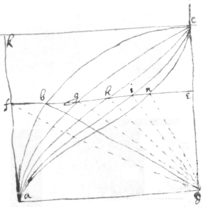abc a circle agc a Parabola. &c. & yt de=x. {illeg} ad∥fe=1=bd. & yt ye progression in wch ye lines fe, be, ge, he, ie, ne &c proceedes is $1.\sqrt{1-xx}$. $1-xx$. $\overline{1-xx}\sqrt{1-xx}$. $1-2xx+{x}^{4}$ . $\overline{1-2xx+{x}^{4}}\sqrt{1-xx}$. $1-3xx+3{x}^{4}-{x}^{6}$. &c. Then will their areas fade, bade, gade, hade, {e}|i|ade, &c be in this progression. $x.\ast .x-\frac{xxx}{3}.\ast .x-\frac{2}{3}{x}^{3}+\frac{1}{5}{x}^{5}.\ast .$ $x-\frac{3{x}^{3}}{3}+\frac{3{x}^{5}}{5}-\frac{{x}^{7}}{7}.\ast .x-\frac{4{x}^{3}}{3}+\frac{6{x}^{5}}{5}-\frac{4{x}^{7}}{7}+\frac{{x}^{9}}{9}$. &c: as in this table following in wch ye intermediate termes are inserted. The property of which table is yt ye $\begin{array}{rrrrrrrrrrrrrrrrr}x& ×& 1.& 1.& 1.& 1.& 1.& 1.& 1.& 1.& 1.& 1.& 1.& 1.& 1.& 1.& 1.\\ -\frac{{x}^{3}}{3}& ×& -1.& -\frac{1}{2}.& 0.& \frac{1}{2}.& 1.& \frac{3}{2}.& 2.& \frac{5}{2}.& 3.& \frac{7}{2}.& 4.& \frac{9}{2}.& 5.& \frac{11}{2}.& 6.\\ \frac{{x}^{5}}{5}& ×& 1.& \frac{3}{8}.& 0.& -\frac{1}{8}.& 0.& \frac{3}{8}.& 1.& \frac{15}{8}.& 3.& \frac{35}{8}.& 6.& \frac{63}{8}.& 10.& \frac{99}{8}.& 15.\\ -\frac{{x}^{7}}{7}& ×& -1.& -\frac{5}{16}.& 0.& \frac{3}{48}.& 0.& -\frac{1}{16}.& 0.& \frac{5}{16}.& 1.& \frac{35}{16}.& 4.& \frac{105}{16}.& 10.& \frac{231}{16}.& 20.\\ \frac{{x}^{9}}{9}& ×& 1.& +\frac{35}{128}.& 0.& -\frac{15}{384}.& 0.& \frac{3}{128}.& 0.& -\frac{5}{128}.& 0.& \frac{35}{128}& 1.& \frac{315}{128}.& 5.& \frac{1155}{128}.& 15.\end{array}$ sume of any figure & ye figure above it is equall to ye figure next after it save one. Also ye pro numerall progressions are of these formes.

 a. a. a. a. b. a+b. 2a+b. 3a+b. c. b+c. a+2b+c. 3a+3b+c. & d. c+d. b+2c+d. a+3b+3c+d. e. d+e c+2d+e. b+3c+3d+e.

Where ye calculation of ye intermediate termes may bee easily performed. The area abed depends upon ye 4th Collume $1.\frac{1}{2}.-\frac{1}{8}.\frac{3}{48}$ &c: (wch progression may bee continued at pleasure by ye helpe of this rule $\begin{array}{ccccccccccccccccccc}0& ×& 1& ×& -1& ×& 3& ×& -5& ×& 7& ×& -9& ×& 11& ×& -13& ×& 15\\ 0& ×& 2& ×& -4& ×& 6& ×& -8& ×& 10& ×& -12& ×& -14& ×& 16& ×& -18\end{array}$ &c.) Whereby it may appeare yt, what ever ye sine de=x is, {illeg} ye area abed is $x-\frac{{x}^{3}}{6}-\frac{{x}^{5}}{40}-\frac{{x}^{7}}{112}-\frac{5{x}^{9}}{1152}-\frac{7{x}^{11}}{2816}-\frac{21{x}^{13}}{13312}-\frac{11{x}^{15}}{10240}$ &c. (& ye area afb is $\frac{{x}^{3}}{6}+\frac{{x}^{5}}{40}+\frac{{x}^{7}}{112}$ &c.) Whereby also ye area & angle adb may bee found. The same may bee done thus. ye areas afd, abd, agd, ahd &c are in this progression $\frac{x}{2}.\ast .\frac{x}{2}+\frac{{x}^{3}}{3}.\ast .\frac{x}{2}+\frac{2{x}^{3}}{6}-\frac{3{x}^{5}}{10}.\ast .\frac{x}{2}+\frac{3{x}^{3}}{6}-\frac{9{x}^{5}}{10}+\frac{5{x}^{7}}{14}.\ast .\frac{x}{2}+\frac{4{x}^{3}}{6}-\frac{18{x}^{5}}{10}+\frac{40{x}^{7}}{14}-\frac{7{x}^{9}}{18}$ . &c As in this following Table $\begin{array}{rrrrrrrr}\frac{1}{2}x& ×& 1.& 1.& 1.& 1.& 1.& 1\\ \frac{1}{6}{x}^{3}& ×& \frac{1}{2}& 1.& \frac{3}{2}.& 2.& \frac{5}{2}.& 3\\ -\frac{3}{10}{x}^{5}& ×& -\frac{1}{8}.& 0.& \frac{3}{8}.& 1.& \frac{15}{8}.& 3\\ \frac{5}{14}{x}^{7}& ×& \frac{1}{16}.& 0.& -\frac{1}{16}.& 0.& \frac{5}{16}.& 1\\ -\frac{7}{18}{x}^{9}& ×& -\frac{5}{128}.& 0.& \frac{3}{128}.& 0.& -\frac{5}{128}.& 0\\ & & =& =& =& =& =& \\ & & abd& agd& ahd& aid& and& \end{array}$ By wch it may bee perceived yt $abd=\frac{1}{2}x+\frac{1}{12}{x}^{3}+\frac{3}{80}{x}^{5}+\frac{5}{224}{x}^{7}+\frac{35{x}^{9}}{2304}+\frac{63{x}^{11}}{5632}$ . &c. And by this meanes haveing ye area abd, ye (wch ye angle adb gives) \de/ ye sine of ye angle adb may bee found. Corol: If {illeg} \de/=x. & {illeg} $\sqrt{1+xx}=eb$ . yn abc is an Hyperbola. & its area {illeg} dabe is $x+\frac{{x}^{3}}{6}-\frac{{x}^{5}}{40}+\frac{{x}^{7}}{112}-\frac{5{x}^{9}}{1152}+\frac{7{x}^{11}}{2816}$ . &c.

<73r>1. Fiat bd∥ad, et ducantur rectæ ab, gd, ag, bd: Conicarum portiones bas, gdt, erunt æquales, item et ga|s|b, bdtg. \(fig 1.2)/ Bisectis enim bg, ad, in punctis r, et e; et ducto ce|r|e; erunt brg aed ordinatim applicatæ ad diamet{er}|ru|m cure: proinde portiones reasb=redgtg, et trapezia reab=redg. Ergo eorum differentiæ bas=gdt. Deinde △abg=dgb (per 37.1. Elem), Ergo portiones gasbv=bdtgv.

Coroll. Hinc pateat modus ducendi tangentes ad Conicas, ignoratis eorum diamet{illeg}|ris|: Duc \A dato/ enim (fig 3) puncto a, duc ac, ab, {&}|et| ijs parallelas bd, cf: linea df erit parallela tant|g|enti ah. Si non, fiat ae∥df, \et duc de, ad, af, cg;/ et erit portio ecd=abf{illeg}t ecd=abf=bac=aecd, qd est impos:

Porro {illeg} fiat dn=nf, et duc \{illeg}/ ank; fiat am=mk, et duc d pmq. Erunt ak, pq diametri.

Diametro av describatur circulus abcdv. Et centro a,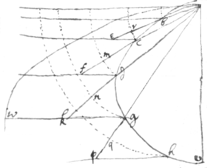describantur circuli cr, dm, gn, hq. et a punctis c, d, g, h, duc ce, df, gk, hp, perpendiculares ad diametrum av; & duc abe, acf, adk, agp. In triangulis gqh, pqh &c: angulus hgp=hpq & ∠gqh=∠pqh=recto. Ergo, si ponantur trianguli qgh, qph esse infinitè parvi, ut latera hg, hq sit rectæ; erunt similes et æquales, poinde {sic} gq=pq. Hinc omnes lineæ gp, dk, cf, be, & sunt duplices linearum gq, dn cm, br, &c. hoc est longitudo Trochoid: est an est duplex longit: rectæ ag.

<74r>

## De Gravitate Conicarum.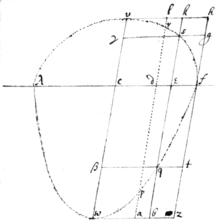Sit λvf Ellipsis, et λwf Parabola. Ita nempe, ut λd vocatâ x; ordinatim applicata d{illeg} sit $dr=\sqrt{rx-\frac{rxx}{q}}$ , & $dp=\frac{rx}{2}-\frac{rxx}{2q}$ . Fiat λc=cf= $\frac{1}{2}q$ . & d{illeg}w∥hfz∥kb∥kb∥rp, & vh∥wz∥γg∥βt, tangentes vel sec{illeg} sese aut curvas in punctis v, l, k, h, r, γ, s, g, c, d, e, f, β, q, t, p, w, a, b, z.

Dico quòd, posito λf axe gravitatis, pondus parallelogrammes|i| vkec est ad pondus portionis vsec, sicut parallelogram w|v|bec ad portionem wqec.

Ponatur enim pondus lineæ id esse $\frac{1}{2}ld×ld$ siv dr esse $\frac{1}{2}dr×dr$ sive $\frac{rx}{2}-\frac{rxx}{2q}$ , hoc uest dp. Erit pondus omniu lineæ|aru| dr in superficie vsec contentarum, hoc est pondus superficiei vsec, æquale|i| superficiei wqec. Et eâdem ratione pondus ▱mi vkec erit mum wbec. Q.E.D.

Coroll. Pondus portionis vks est wbq, est portionis vsγ est wqβ, et portionis esf est eqf, & portionis fsg est fqt &c. Quæ omnia dat quadratura Parabolæ, est enim 3βwq=2βwbq, &c.

Eâdem ratione gravitas cujuslibet portionis Hyperbolæ \cognoscatur/, modò axis gravitatis transeat per centrum Hyperbolæ. Et si quævis plana superficies conicis sectionibus ità terminetur, ut omnia conicarum centra sint in eâdem rectâ lineâ: gravitas istius superficiei inv{illeg}eniri potest, positâ rectâ gravitatis axi. Deni, centrum gravitatis cujusvis planæ \& finitæ/ superficiei conicis sectionibus \itâ|a|/ terminatæ inveniri potest, datâ quantitate istius superficiei, & vice versâ, modò centrum gravitatis aixi {sic} gravitatis non coïncidat.

Sint ac, af Assymptota Hyperbolæ gc, in infinitu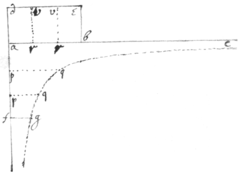versus c continuatæ. Duc de=ab=af. & $eb=ad=\sqrt{af×fg}$ . |& fg∥ac.| Dico qd. Parallelogramu ae, & superficies a{illeg}fgc æquiponderant circa axim abc: Etsi superficies aefg afgc versus c sit longitudine & quantitate infinita, & non habet centrum gravitatis.

fiat enim ar=ap. & duc pq∥ab. & vr∥ad. et sit pq×$\frac{1}{2}$ap gravitas lineæ pq, erit vr×$\frac{1}{2}$vr gravitas lineæ vr (nom ∠vrb=∠apq). sed $pq×\frac{1}{2}pq=\frac{af×fg}{2}=$ $=\frac{ad×ad}{2}=vr×\frac{1}{2}vr$ . æquiponderant ergo lineæ pq, vr. Sed numerus linearum pq in superficie acgf est æqualis numero linearum vr in parallelogrammo ae nam af=ab, ergo ae & ag æquiponderant.

Add to the end of the Paragraph. See the mystery. \In the first instance from the series $1+\frac{1}{2}+\frac{1}{3}$ &c subduct $\frac{1}{2}+\frac{1}{3}+$ &c. & then will remain/ $1+\frac{1}{2}+\frac{1}{3}+\frac{1}{4}+\frac{1}{5}$ &c $-\frac{1}{2}-\frac{1}{3}-\frac{1}{4}-\frac{1}{5}$ &c=1= |will remain| $1-\frac{1}{2}+\frac{1}{2}-\frac{1}{3}+\frac{1}{3}-\frac{1}{4}+\frac{1}{4}-\frac{1}{5}\text{&c}=\frac{1}{1×2}+\frac{1}{2×3}+\frac{1}{3×4}+\frac{1}{4×5}$ &c. And so of the rest Or take all the terms but the three first & there will remain $\frac{11}{6}=\frac{3}{1×4}+\frac{3}{2×5}+\frac{3}{3×6}$ &c Or from this series take all the termes b $\frac{1}{1}+\frac{1}{3}+\frac{1}{5}+\frac{1}{7}+\frac{1}{9}$ &c take all the termes but the termes but the first & there will remain $1=\frac{2}{1×3}+\frac{2}{3×5}+\frac{2}{5×7}+\frac{2}{7×9}$ &c.

$\begin{array}{cc}\begin{array}{rrr}23883.12& 107474& 19902.6\\ 214948.08& & 1791234\\ & & 895617\end{array}& \begin{array}{rr}3654×76& 21924\\ & 25578\\ & 277704\end{array}\end{array}$

<75r>

## Problems of Curves.

Probl. 1.

To draw Tangents or Perpendiculars to any given point of a given Curve (V B) (Sup. VA=x, AB=y)

Put the equation of the Curve=0, multip. by this Progrn. $+.0.\frac{y}{x}.\frac{2y}{x}.\frac{3y}{x}$ &c. according to the dimensions of x for a numerator, change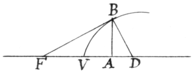the signs and multip. by this Progr. $0.\frac{1}{y}.\frac{2}{y}.\frac{3}{y}$ &c for a Denominator, which shall make the Fraction designing AD to be taken forwards from A, if affirmative, otherwise, backwards.

Examp. 1. If a+bxy−xxy+y3=0, then $\frac{byy-2xyy}{bx-xx+3yy}=AD=v$ (the Subperpendicular.

Coroll.

Hence for AF (the Subtangent) multiply by the Dimensions of y for a Numerator & by this Progr. $0.\frac{1}{x}.$ $\frac{2}{x}.\frac{3}{x}$ &c according to the Dimension of x for a Denominator; thus results $\frac{bx-xx+3yy}{-b+2x}=AF=t$ .

Prob. 2d..

From a given point H to draw a Tangent (HB) to any Curve (VB)

Sup. VG=p, GH=q and AF=t and it is y−q∶x+p∷y∶ t. Th. $\frac{p{q}^{y}+xy}{y-q}=t$ , or ty−tq=xy+py. If into this you substitute the value of t (found ꝑ Prob. 1.) you will have the nature of a Line wch described will cut the propounded Curve in the desired Tangt. points.

Exa. Thus substituting $\frac{bx-ax+3yy}{-b+xx}$ for t, there comes bxy−xxy+3y3−bqx+qx2−3qy2=2x2y+2pxy{illeg} {illeg} −b{illeg}x4+bpy=0, \the Curve to be described/ *|* But note that this may be reduced to a simpler line by adding or substracting the nature of the given line, viz. ordering this results, it is 3y3−3x2y+2bxy−2pay+qxx−3qyy−bqx+bpy=0.| from whence substracting y3−xxy +bxy+a\thrice/ there rests −bxy−2pxy+qx2−3qyy−bqx+bqy −3a=0, a Conic section wch described will cut the Curve in the desired tangt poin{illeg}ts.

Thus if $ax+\frac{b}{a}xx-yy=0$ , then $\frac{2yy}{a+\frac{2b}{a}x}=t=\frac{py+xy}{y-q}$ <75v> or $2yy-2qy=\frac{2b}{a}xx+ax+\frac{2b}{a}px+ap$ & substracting $ax+\frac{b}{a}xx-yy=0$ twice (viz so often as the Curve has dimensions) there comes $\frac{2bpx}{a}-ax+2qy+ap=0$ , a strait line wch drawn will cut ye Curve in the points desired & so of the rest.

Where note that this Problem in any Curve is al ever solvable by a line of an inferior degree. And also that a line drawn from a given point may touch a Curve of two Dimensions in 1×2 points, of three in 2×3 points of 4, in 3×4 pts. &c unless it be polar &c.

Probl. 3.

From a given point (C) to draw a Perpend{i}r. (CB) to any Curve (VB)

Make VE=r, EC=s and AD=v so is y−s∶r−{s}\x/y∶v. or $\frac{ry-xy}{y-s}=v$ put this valor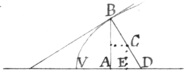of v in the room of v found ꝑ Prob. 1. And and you have a Curve wch described will cut the propounded Curve in the points to wch the perpendiculr may be drawn [but ever try if by means of the nature of the given Curve you can reduce this resulting to any simpler form or degree.

Exa. If ax+$\frac{b}{a}$xx−yy=0, then $\frac{1}{2}$a+$\frac{b}{a}$x=v, or ry−xy=$\frac{1}{2}$y−$\frac{1}{2}$as+$\frac{b}{a}$xy−$\frac{b}{a}$sx, wch appears not reducible to a simpler form.

Yet it may be worth while to try if it may be solved by a Circle by assuming d+ex+fy=xx+yy and comparing these 3 equations of the given Conick, found {illeg} Hyperbola and assum'd Circle to find d, e & f wch if they be found by plane Geometry that Circle described will cut the Curve in the defined points. This, I say, might be tried but it would be found impossible (unless the Conic be a Parabola) because there are 4 given points thró wch the Circle must pass, wch make the Probl. contradicting, since 3 are {now} to determine a Circle.

This might be done by the Parabola, but the Hyperbola falling in so naturally, and being as easily described, 'tis not worth the while.

What is said of the Conics may be applied to any other Curves.

From hence it appears that this Probl. is ever solvable by a Curve of the same degree and sometimes ꝑhaps by a Curve of an inferior Degree.

<76r>

Also from a given point may be drawn so many ꝑpendicr, as the square of the Curves Dimensions, unless some tt extraordry sum out to infinity, or two ts come together to make it polar.

Probl. 4.

To find the Points where the Curve has a given inclination to the Basis.

Supp. FA∶AB or AB∶AD∷m∶n.Then $\frac{my}{n}=t$ . Put this eql. to the value of t found by Prob. 1. & you have a Curve wch describ'd will cut the given Curve in the desired points.

But (if you can) reduce it th{illeg}s\us/ if $ax+\frac{b}{a}xx-yy=0$ , then $\frac{2yy}{a+\frac{2b}{a}x}=t=\frac{my}{n}$ , or $2ny=am+\frac{2mb}{a}x$ . the like in other \cases./

Hence Conics have 1×2 points, those of 3 Dimensions 2×3 points, of 4 Dimens. 3×4. &c. will satisfy this Probl, & its ever soluble by a line of an inferior Degree.

Probl. 5.

From a given point (C) to draw a line CB wch shall cut any given Curve in a given Angle.

Supp. BD the ꝑpendr to ye desir'd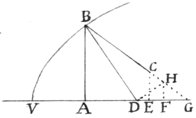point B & from the point D raise the ꝑpendr. DH & let fall HF⊥ AE, producing BC & AE to G. Let AD=v, VE=r, EC=s and (ye ∠ CBD being given) supp. BD∶DH∷m∶n.

Now the △s BHD & DFH being alike, 'tis m∶n∷AB∶ DF∷AD∶FH. Th. $DF=\frac{ny}{m}$ & $\frac{rs-xs}{y-s}=EG$ & $\frac{rm-2nv}{my-ms}=FG$. Lastely since VE+EG=VG=VA+AD+DF +FG there results $r+\frac{rs-xs}{y-s}=x+v+\frac{ny}{m}+\frac{rnv-xnv}{my-ms}$ wch reduced is $\frac{mry-mxy+nsy-nyy}{my-ms+nr-nx}=v$ (mr+n put mr+ns =k & nr−ms=l to shorten the terms) this I put equal to the valor of v found as before, and there results a Curve wch described cuts ye given Curve in the points desired, to wch lines are to be drawn from C that may cut it in the Angles desired.

Exa. If dd+$\frac{a}{b}$xx−yy=0, then is $\frac{a}{b}$x={illeg} \v/, and so mry −mxy+nsy−nyy= $\frac{ma}{b}xy-\frac{mas}{b}x+\frac{nar}{b}x-\frac{na}{b}xx$ , which is a Conic section passing thrò the Centre of the given Curve. And <76v> it may be made an Hyperbola constantly by substituting dd for yy−$\frac{a}{b}$2x. For the result will be $dd+\frac{nar}{b}x-\frac{mas}{b}x-mry-nsy+nxy-\frac{ma}{b}2y=0$.

Hence it appears that this Problem may ever be solved by a Curve of the same sort, and sometimes ꝑhaps by one of an inferior.

And also that from a given point to any Curve so many lines may be drawn in a given Angle as the sqr. of its Dimensions abating those that are imaginary, or coincident to the Pole, or should be drawn where some ꝑts of the Curve vanishes ad infinitum.